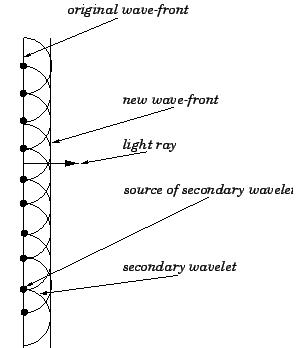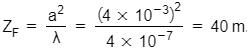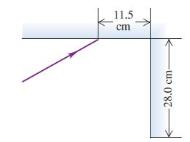Courses

# Test: Wave Optics (CBSE Level With Solutions)

## 30 Questions MCQ Test Physics Class 12 | Test: Wave Optics (CBSE Level With Solutions)

Description
This mock test of Test: Wave Optics (CBSE Level With Solutions) for Class 12 helps you for every Class 12 entrance exam. This contains 30 Multiple Choice Questions for Class 12 Test: Wave Optics (CBSE Level With Solutions) (mcq) to study with solutions a complete question bank. The solved questions answers in this Test: Wave Optics (CBSE Level With Solutions) quiz give you a good mix of easy questions and tough questions. Class 12 students definitely take this Test: Wave Optics (CBSE Level With Solutions) exercise for a better result in the exam. You can find other Test: Wave Optics (CBSE Level With Solutions) extra questions, long questions & short questions for Class 12 on EduRev as well by searching above.
QUESTION: 1

### Is light a particle or a wave?

Solution:

Explanation:explanation none

QUESTION: 2

Solution:
QUESTION: 3

### The angle of incidence at which the reflected beam is fully polarized is called

Solution:
QUESTION: 4

The refractive index of glass is 1.5 which of the two colors red and violet travels slower in a glass prism?

Solution:

(a) Speed of light in glass is c/μ=2×108m/s

(b) The speed of light in glass is not independent of the colour of light. The refractive index of a violet component of white light is greater than the refractive index of a red component. Hence, the speed of violet light is less than the speed of red light in glass. Hence, violet light travels slower than red light in a glass prism.

QUESTION: 5

In a young’s double slit experiment, the central bright fringe can be identified by

Solution:
QUESTION: 6

The propagation of light is best described by,

Solution:
QUESTION: 7

In general the term diffraction is used

Solution:
QUESTION: 8

Shape of the wave front of light diverging from a point source is

Solution:
QUESTION: 9

Emission and absorption is best described by,

Solution:
QUESTION: 10

In a Young’s double-slit experiment, the slits are separated by 0.28 mm and the screen is placed 1.4 m away. The distance between the central bright fringe and the fourth bright fringe is measured to be 1.2 cm. Determine the wavelength of light used in the experiment

Solution:

Both emission and absorption techniques can be used to get the same information about the energy levels of an atom. The dark lines correspond to the frequencies of light that have been absorbed by the gas. As the photons of light are absorbed by electrons, the electrons move into higher energy levels.

QUESTION: 11

Diffraction also refers to

Solution:
QUESTION: 12

Shape of the wave front of light emerging out of a convex lens when a point source is placed at its focus.

Solution:
QUESTION: 13

A beam of light consisting of two wavelengths, 650 nm and 520 nm, is used to obtain interference fringes in a Young’s double-slit experiment. Find the distance of the third bright fringe on the screen from the central maximum for wavelength 650 nm.

Solution:

Solution :- Let at liner distance 'y' from center of screen the bright fringes due to both wavelength coincides. Let the n1 number of bright fringe with wevelength λ1 coincides with n2 number of bright fringe with wavelength λ2

We can write

y = n1​β1 = n2β2

n1 = λ1D/d                = n2​Dλ2/d                 or n1λ1=n2λ2

​Also at first position of coincide the th bright fringe of one will coincide with (n+1)th bright fringe of other.

If λ2<λ1,

So, then n2>n1

and n2 = n1+1

Using equation (ii) in equation (i)

n1λ1=(n1+1)λ2

​n1(650)×10^−9 = (n1+1)520×10^−9

65n1 = 52n1 + 52 or 12n1 = 52or n1=3

Thus, y = n1β1 = 3[(6.5×10^−7)(1.2)]/2×10^−3 = 1.17×10^−.3

m=1.17mm

QUESTION: 14

A beam of light has a wavelength of 650 nm in vacuum. What is the wavelength of these waves in the liquid whose index of refraction at this wavelength is 1.47?

Solution:
QUESTION: 15

wave front is

Solution:
QUESTION: 16

A beam of light consisting of two wavelengths, 650 nm and 520 nm, is used to obtain interference fringes in a Young’s double-slit experiment. What is the least distance from the central maximum where the bright fringes due to both the wavelengths coincide?

Solution:

Fringe width of 650nm wavelength is 650D/d and that of 520nm wavelength is 520D/d
Where D is distance between slits and screen and d is distance between screens.
The common constructive pattern will be seen at the minimum possible common multiple of both the wavelengths.

QUESTION: 17

A parallel beam of light in air makes an angle of 47.5 with the surface of a glass plate having a refractive index of 1.66. What is the angle between the reflected part of the beam and the surface of the glass?

Solution:

The angle of incidence = 90 - 47.5 = 42.5
Now by snells’ laws we get
sin r = sin 42.5 / 1.66
Thus we get r = 24.01
Thus the angle with surface = 90 -24.01 = 65.99

QUESTION: 18

Shape of the wave front of portion of the wave front of light from a distant star intercepted by the Earth

Solution:
QUESTION: 19

Which of the following phenomenon cannot be explained by diffraction?

Solution:
QUESTION: 20

According to Huygens principle

Solution:
Every point on a wave-front may be considered a source of secondary spherical wavelets which spread out in the forward direction at the speed of light. The new wave-front is the tangential surface to all of these secondary wavelets.

According to Huygens' principle, a plane light wave propagates though free space at the speed of light, c. The light rays associated with this wave-front propagate in straight-lines. It is also fairly straightforward to account for the laws of reflection and refraction using Huygens' principle.QUESTION: 21

For distances much greater than Fresnel distance ZF

Solution:
QUESTION: 22

Estimate the distance for which ray optics is good approximation for an aperture of 4 mm and wavelength 400 nm.

Solution:

Here,
Aperture, a = 4 mm = 4 × 10-3 m
Wavelength, λ = 400 nm = 400 × 10-9 m = 4 × 10-7 m

Ray optics is good approximation upto distances equal to Fresnel's distance (ZF).
Fresnel's distance is given by,QUESTION: 23

The critical angle for total internal reflection at a liquid–air interface is 42.5 If a ray of light traveling in air has an angle of incidence at the interface of 35 what angle does the refracted ray in the liquid make with the normal?

Solution:
QUESTION: 24

According to Huygens construction relation between old and new wave fronts is

Solution:
QUESTION: 25

We say that a light wave is linearly polarized in y direction if

Solution:
QUESTION: 26

A parallel beam of light of wavelength 500 nm falls on a narrow slit and the resulting diffraction pattern is observed on a screen 1 m away. It is observed that the first minimum is at a distance of 2.5 mm from the centre of the screen. Find the width of the slit.

Solution:

Wavelength of light beam, λ

Distance of the screen from the slit, D=1m

For first minima, n=1

Distance between the slits is d

Distance of the first minimum from the centre of the screen can be obtained as, x = 2.5mm = 2.5×10−3

Now, nλ = xd/D

⇒ d= nλD/x = 0.2mm

Therefore, the width of the slits is 0.2 mm.

QUESTION: 27

Relation between ray and wave front is

Solution:
QUESTION: 28

Two plane mirrors intersect at right angles. A laser beam strikes the first of them at a point 11.5 cm from their point of intersection, as shown in figure For what angle of incidence at the first mirror will this ray strike the midpoint of the second mirror (which is 28.0 cm long) after reflecting from the first mirror?Solution:
QUESTION: 29

Two sources of light are coherent if they have

Solution:
QUESTION: 30

If we have two coherent sources S1andS2 vibrating in phase, then for an arbitrary point P constructive interference is observed whenever the path difference is

Solution: